# 22.4: Electrophilic Aromatic Substitution

$$\newcommand{\vecs}{\overset { \rightharpoonup} {\mathbf{#1}} }$$ $$\newcommand{\vecd}{\overset{-\!-\!\rightharpoonup}{\vphantom{a}\smash {#1}}}$$$$\newcommand{\id}{\mathrm{id}}$$ $$\newcommand{\Span}{\mathrm{span}}$$ $$\newcommand{\kernel}{\mathrm{null}\,}$$ $$\newcommand{\range}{\mathrm{range}\,}$$ $$\newcommand{\RealPart}{\mathrm{Re}}$$ $$\newcommand{\ImaginaryPart}{\mathrm{Im}}$$ $$\newcommand{\Argument}{\mathrm{Arg}}$$ $$\newcommand{\norm}{\| #1 \|}$$ $$\newcommand{\inner}{\langle #1, #2 \rangle}$$ $$\newcommand{\Span}{\mathrm{span}}$$ $$\newcommand{\id}{\mathrm{id}}$$ $$\newcommand{\Span}{\mathrm{span}}$$ $$\newcommand{\kernel}{\mathrm{null}\,}$$ $$\newcommand{\range}{\mathrm{range}\,}$$ $$\newcommand{\RealPart}{\mathrm{Re}}$$ $$\newcommand{\ImaginaryPart}{\mathrm{Im}}$$ $$\newcommand{\Argument}{\mathrm{Arg}}$$ $$\newcommand{\norm}{\| #1 \|}$$ $$\newcommand{\inner}{\langle #1, #2 \rangle}$$ $$\newcommand{\Span}{\mathrm{span}}$$$$\newcommand{\AA}{\unicode[.8,0]{x212B}}$$

## Scope and Mechanism

In this section we shall be mainly interested in the reactions of arenes that involve attack on the carbon atoms of the aromatic ring. We shall not elaborate now on the reactions of substituent groups around the ring.

The principal types of reactions involving aromatic rings are substitution, addition, and oxidation. Of these, the most common type is electrophilic substitution. A summary of the more important substitution reactions of benzene is given in Figure 22-7. Many of the reagents used to achieve these substitutions will be familiar to you in connection with electrophilic addition reactions to alkenes (e.g., $$\ce{Cl_2}$$, $$\ce{Br_2}$$, $$\ce{H_2SO_4}$$, and $$\ce{HOCl}$$; Section 10-3). Electrophilic addition to alkenes and electrophilic aromatic substitution are both polar, stepwise processes, and the key step for each is attack of an electrophile at carbon to form a cationic intermediate. We may represent this type of reaction by the following general equations, in which the attacking reagent is represented either formally as a cation, $$\ce{X}^\ominus$$, or as a neutral but polarized molecule, $$\overset{\delta \oplus}{\ce{X}}$$---$$\overset{\delta \ominus}{\ce{Y}}$$:

electrophilic aromatic substitution (first step)electrophilic addition to alkenes (first step)The intermediate shown for aromatic substitution no longer has an aromatic structure; rather, it is a cation with four $$\pi$$ electrons delocalized over five carbon nuclei, the sixth carbon being saturated with $$sp^3$$-hybrid bonds. It may be formulated in terms of the following contributing structures, which are assumed to contribute essentially equally: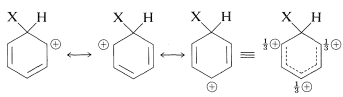The importance of writing the hybrid structure with the partial charges at these three positions will become evident later. This kind of ion is referred to as a $$\sigma$$ complex or a benzenium ion.

The aromatic ring is regenerated from this cationic intermediate by loss of a proton from the $$sp^3$$-hybridized carbon. The electron pair of this $$\ce{C-H}$$ bond then becomes part of the aromatic $$\pi$$-electron system and a substitution product of benzene, $$\ce{C_6H_5X}$$, is formed.

electrophilic aromatic substitution (second step)The gain in stabilization attendant on regeneration of the aromatic ring is sufficiently advantageous that this, rather than combination of the cation with $$\ce{Y}^\ominus$$, normally is the favored course of reaction. Herein lies the difference between aromatic substitution and alkene addition. In the case of alkenes there usually is no substantial resonance energy to be gained by loss of a proton from the intermediate, which tends therefore to react by combination with a nucleophilic reagent.

electrophilic addition to alkenes (second step)

$\overset{\oplus}{\ce{C}} \ce{H_2-CH_2X} + \ce{Y}^\ominus \rightarrow \ce{YCH_2-CH_2X}$

## Nature of the Substituting Agent

It is important to realize that in aromatic substitution the actual electrophilic substituting agent, $$\overset{\oplus}{\ce{X}}$$ or $$\overset{\delta \oplus}{\ce{X}}-\overset{\delta \ominus}{\ce{Y}}$$, is not necessarily the reagent that is added to the reaction mixture. For example, nitration in mixtures of nitric and sulfuric acids is not brought about by attack of the nitric acid molecule on the aromatic compound, but by attack of a more electrophilic species, the nitronium ion, $$\ce{NO_2^+}$$. This ion is formed from nitric acid and sulfuric acid according to the following equation:

$\ce{HNO_3} + 2 \ce{H_2SO_4} \rightleftharpoons \ce{NO_2^+} + \ce{H_3O^+} + 2 \ce{HSO_4^-}$

The nitronium ion attacks the aromatic ring to give first a nitrobenzenium ion and then an aromatic nitro compound:In general, the function of a catalyst (which is so often necessary to promote aromatic substitution) is to generate an electrophilic substituting agent from the given reagents. Thus it is necessary to consider carefully for each substitution reaction what the actual substituting agent may be. This problem does not arise to the same degree in electrophilic additions to alkenes, because alkenes are so much more reactive than arenes that the reagents employed (e.g., $$\ce{Br_2}$$, $$\ce{Cl_2}$$, $$\ce{HCl}$$, $$\ce{HOCl}$$, $$\ce{HOBr}$$, $$\ce{H_3O}^\oplus$$) themselves are sufficiently electrophilic to react with alkenes without the aid of a catalyst. In fact, conditions that lead to substitution of arenes, such as nitration in mixtures of nitric and sulfuric acid, often will degrade the carbon skeleton of alkenes.

Now we shall consider the individual substitution reactions listed in Figure 22-1 with regard to the nature of the substituting agent and the utility for synthesis of various classes of aromatic compounds.

## Nitration

The nitronium ion, $$\ce{NO_2^+}$$, is the active nitrating agent in nitric acid-sulfuric acid mixtures. The nitration of methylbenzene (toluene) is a typical example of a nitration that proceeds well using nitric acid in a 1:2 mixture with sulfuric acid. The nitration product is a mixture of 2-, 3-, and 4-nitromethylbenzenes: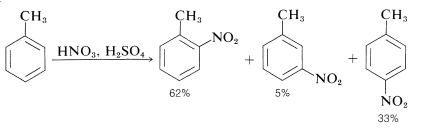The presence of appreciable amounts of water in the reaction mixture is deleterious because water tends to reverse the reaction by which nitronium ion is formed:

$\ce{NO_2^+} + \ce{H_2O} \overset{\ce{HSO_4^-}}{\rightleftharpoons} \ce{HNO_3} + \ce{H_2SO_4}$

For this reason the potency of a nitric-sulfuric acid mixture can be considerably increased by using fuming nitric and fuming sulfuric acids. With such mixtures nitration of relatively unreactive compounds can be achieved. For example, 4-nitromethylbenzene is far less reactive than methylbenzene, but when heated with an excess of nitric acid in fuming sulfuric acid, it can be converted successively to 2,4-dinitromethylbenzene and to 2,4,6-trinitromethylbenzene (TNT):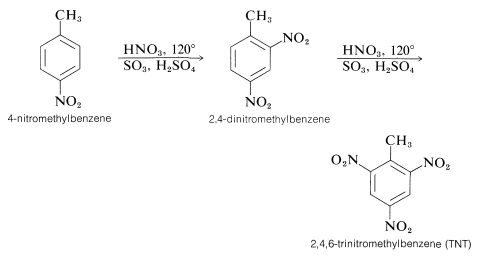There are several interesting features about the nitration reactions thus far discussed. For instance, the conditions required for nitration of 4-nitromethylbenzene would rapidly oxidize an alkene by cleavage of the double bond:Also the mononitration of methylbenzene does not lead to equal amounts of the three possible products. The methyl substituent apparently orients the entering substituent preferentially to the 2 and 4 positions. This aspect of aromatic substitution will be discussed in Section 22-5 in conjunction with the effect of substituents on the reactivity of aromatic compounds.

Some compounds are sufficiently reactive that they can be nitrated with nitric acid in ethanoic acid. Pertinent examples are 1,3,5-trimethylbenzene and naphthalene:Other convenient nitrating reagents are benzoyl nitrate, $$\ce{C_6H_5COONO_2}$$, and ethanoyl nitrate, $$\ce{CH_3COONO_2}$$. These reagents provide a source of $$\ce{NO_2^+}$$ and have some advantage over $$\ce{HNO_3} \cdot \ce{H_2SO_4}$$ mixtures in that they are soluble in organic solvents such as ethanenitrile or nitromethane. Having homogeneous solutions is especially important for kinetic studies of nitration. The reagents usually are prepared in solution as required from the corresponding acyl chloride and silver nitrate or from the acid anhydride and nitric acid. Such reagents are hazardous materials and must be handles with care.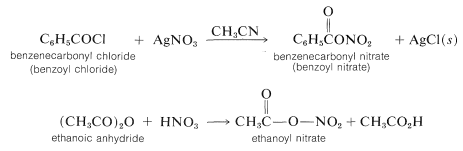Nitronium salts of the type $$\ce{NO_2^+} \ce{X^-}$$ are very powerful nitrating agents. The counterion, $$\ce{X^-}$$, must be non-nucleophilic and usually is fluoroborate, $$\ce{BF_4^-}$$ or $$\ce{SbF_4^-}$$:## Halogenation

To some degree we have oversimplified electrophilic substitution by neglecting the possible role of the 1:1 charge-transfer complexes that most electrophiles form with arenes (see Section 10-3C for discussion of analogous complexes of alkenes):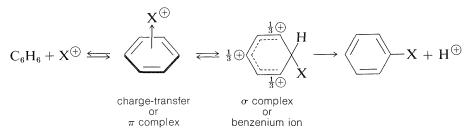With halogens, especially iodine, complex formation is visually evident from the color of solutions of the halogen in arenes. Although complex formation may assist substitution by bringing the halogen and arene in close proximity, substitution does not necessarily occur. A catalyst usually is required, and the catalysts most frequently used are metal halides that are capable of accepting electrons (i.e., Lewis acids such as $$\ce{FeBr_3}$$, $$\ce{AlCl_3}$$, and $$\ce{ZnCl_2}$$). Their catalytic activity may be attributed to their ability to polarize the halogen-halogen bond in the following way:

$\overset{\delta \oplus}{\ce{Br}} \cdots \overset{\delta \ominus}{\ce{Br}} \cdots \ce{FeBr_3}$

The positive end of the dipole attacks the aromatic compound while the negative end is complexed with the catalyst. We can represent the reaction sequence as follows, with the slow step being formation of a $$\sigma$$ bond between $$\ce{Br}^\oplus$$ and the aromatic ring:The order of reactivity of the halogens is $$\ce{F_2} > \ce{Cl_2} > \ce{Br_2} > \ce{I_2}$$. Fluorine is too reactive to be of practical use for the preparation of aromatic fluorine compounds and indirect methods are necessary (see Section 23-10B). Iodine usually is unreactive. It has been stated that iodination fails because the reaction is reversed as the result of the reducing properties of the hydrogen iodide that is formed:

$\ce{C_6H_6} + \ce{I_2} \overset{\rightarrow}{\longleftarrow} \ce{C_6H_5I} + \ce{HI}$

This view is not correct because, as Kekule himself showed, iodobenzene is not reduced by hydroiodic acid except at rather high temperatures.

The way to achieve direct iodination in the absence of powerful activating substituent groups is to convert molecular iodine to some more active species (perhaps $$\ce{H_2OI}^\oplus$$ or $$\ce{I}^\oplus$$) with an oxidizing agent such as nitric acid or hydrogen peroxide:

\begin{align} \ce{I_2} + 4 \ce{HNO_3} &\rightarrow 2 \ce{H_2O-I^+} + 2 \ce{NO_2} + 2 \ce{NO_3^-} \\ \ce{I_2} + \ce{H_2O_2} + 2 \ce{H^+} &\rightarrow 2 \ce{H_2OI^+} \end{align}

With combinations of this kind good yields of iodination products are obtained:Halogen substitution reactions with chlorine or bromine must be carried out with adequate protection from strong light. If such precautions are not taken, an alkylbenzene will react rapidly with halogen by a photochemical process to substitute a hydrogen of the alkyl group rather than of the aromatic ring. The reaction has a light-induced, radical-chain mechanism of the kind discussed for the chlorination of propene (Section 14-3A). Thus methylbenzene reacts with bromine when illuminated to give phenylmethyl bromide; but when light is excluded and a Lewis acid catalyst is present, substitution occurs to give principally the 2- and 4-bromomethylbenzenes. Much less of the 3-bromomethylbenzene is formed:Benzene itself can be induced to add halogens on strong irradiation to give polyhalocyclohexanes (see Sections 21-3A and 22-9C):

## Alkylation

An important method of synthesis of alkylbenzenes utilizes an alkyl halide as the alkylating agent and a metal halide, usually aluminum chloride, as catalyst: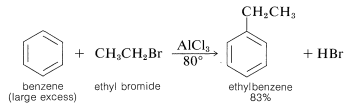This class of reaction is called Friedel-Crafts alkylation in honor of its discoverers, C. Friedel (a French chemist) and J. M. Crafts (an American chemist). The metal-halide catalyst functions much as it does in halogenation reactions to provide a source of a positive substituting agent, which in this case is a carbocation:Alkylation is not restricted to alkyl halides; alcohols and alkenes may be used to advantage in the presence of acidic catalysts such as $$\ce{H_3PO_4}$$, $$\ce{H_2SO_4}$$, $$\ce{HF}$$, $$\ce{BF_3}$$, or $$\ce{HF-BF_3}$$. Ethylbenzene is made commercially from benzene and ethene using phosphoric acid as the catalyst. Isopropylbenzene is made similarly from benzene and propene: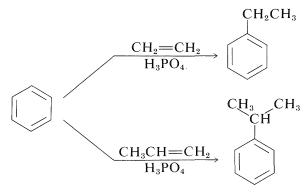Under these conditions the carbocation, which is the active substituting agent, is generated by protonation of the alkene:

\begin{align} \ce{CH_2=CH_2} + \ce{H^+} &\rightleftharpoons \ce{CH_3CH_2^+} \\ \ce{CH_3CH=CH_2} + \ce{H^+} &\rightleftharpoons \ce{CH_3} \overset{+}{\ce{C}} \ce{HCH_3} \end{align}

With alcohols the electrophile can be formed by initial protonation by the acid catalyst and subsequent cleavage to a carbocation:### Limitations of Alkylation Reactions

Polysubstitution

There are several factors that limit the usefulness of alkylation reactions. First, it may be difficult to limit reaction to monosubstitution because introduction of one alkyl substituent tends to activate the ring towards a second substitution (see Section 22-5). Therefore, to obtain reasonable yields of a monoalkylbenzene, it usually is necessary to use a large excess relative to the alkylating agent: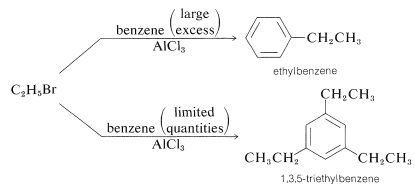Rearrangement of the alkylating agent

A second limitation is the penchant for the alkylating reagent to give rearrangement products. As an example, the alkylation of benzene with 1-chloropropane leads to a mixture of propylbenzene and isopropylbenzene. We may write the reaction as first involving formation of a propyl cation, which is a primary carbocation:

$\ce{CH_3CH_2CH_2Cl} + \ce{AlCl_3} \rightarrow \ce{CH_3CH_2CH_2^+} + \overset{-}{\ce{Al}} \ce{Cl_4}$

This ion either can alkylate benzene to give propylbenzene,

$\ce{C_6H_6} + \ce{CH_3CH_2CH_2^+} \rightarrow \ce{C_6H_5CH_2CH_2CH_3} + \ce{H^+}$

or it can rearrange to a more stable secondary ion by the transfer of a hydrogen from a neighboring carbon together with its bonding electron pair (i.e., 1,2-hydride shift). The positive charge is thereby transferred from $$\ce{C_1}$$ to $$\ce{C_2}$$: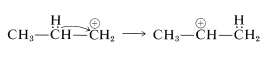Alkylation of benzene with the isopropyl cation then produces isopropylbenzene:

$\ce{C_6H_6} + \ce{CH_3} \overset{\oplus}{\ce{C}} \ce{HCH_3} \rightarrow \ce{C_6H_5CH(CH_3)_2} + \ce{H}^\oplus$

Rearrangements of this type involving carbocation intermediates often occur in Friedel-Crafts alkylations with primary and secondary alkyl groups larger than $$\ce{C_2}$$ and $$\ce{C_3}$$. Related carbocation rearrangements are discussed in Sections 8-9B and 15-5E.

Rearrangement of products

Further complications arise from the fact that the alkylation reactions sometimes are under equilibrium control rather than kinetic control. Products often isomerize and disproportionate, particularly in the presence of large amounts of catalyst. Thus 1,2- and 1,4-dimethylbenzenes (ortho- and para-xylenes) are converted by large amounts of Friedel-Crafts catalysts into 1,3-dimethylbenzene (meta-xylene):Ethylbenzene disproportionates under the influence of excess $$\ce{HF-BF_3}$$ to benzene and 1,3-diethylbenzene: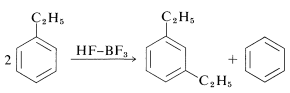## Acylation

Acylation and alkylation of arenes are closely related. Both reactions were developed as the result of the collaboration between Friedel and Crafts, in 1877. The acylation reaction introduces an acyl group, $$\ce{RCO}$$, into an aromatic ring and the product is an aryl ketone: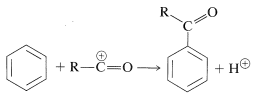The acylating reagents commonly used are carboxylic acid halides, $$\ce{RCOCl}$$, anhydrides, $$\ce{(RCO)_2O}$$, or the acid itself, $$\ce{RCO_2H}$$. A strong proton or other Lewis-acid catalyst is essential. The catalyst functions to generate the acyl cation:The catalyst most commonly used with acyl halides and anhydrides is aluminum chloride:Acylation differs from alkylation in that the reaction usually is carried out in a solvent, commonly carbon disulfide, $$\ce{CS_2}$$, or nitrobenzene. Furthermore, acylation requires more catalyst than alkylation, because much of the catalyst is tied up and inactivated by complex formation with the product ketone:Unlike alkylation, acylation is controlled easily to give monosubstitution, because once an acyl group is attached to a benzene ring, it is not possible to introduce a second acyl group into the same ring. Because of this, a convenient synthesis of alkylbenzenes starts with acylation, followed by reduction of the carbonyl group with zinc and hydrochloric acid (Section 16-6). For example, propylbenzene is prepared best by this two-step route because, as we have noted, the direct alkylation of benzene with propyl chloride produces considerable amounts of isopropylbenzene and polysubstitution products:In the acylation of alkylbenzene the product almost always is the para isomer. The synthesis of (4-tert-butylphenyl)ethanone illustrates this as well as the sequential use of alkylation and acylation reactions:Chemists are inclined to give names to reactions that associate them either with their discoverers or with the products they give. This practice can be confusing because many named reactions (or "name reactions") which once were thought to be quite unrelated, have turned out to have very similar mechanisms. Thus we have two closely related acylation reactions: one is the Friedel-Crafts ketone synthesis, in which the electrophile is $$\ce{R} \ce{-} \overset{\oplus}{\ce{C}} \ce{=O}$$; and the other is the Gattermann-Koch aldehyde synthesis, in which the electrophile is $$\ce{H} \ce{-} \overset{\oplus}{\ce{C}} \ce{=O}$$:The latter reaction utilizes carbon monoxide and $$\ce{HCl}$$ under pressure in the presence of aluminum chloride. The electrophile may be considered to be formed as follows:

$\ce{C=O} + \ce{HCl} + \ce{AlCl_3} \rightleftharpoons \ce{H} \ce{-} \overset{\oplus}{\ce{C}} \ce{=O} + \overset{\ominus}{\ce{Al}} \ce{Cl_4}$

## Sulfonation

Substitution of the sulfonic acid $$\left( \ce{-SO_3H} \right)$$ group for a hydrogen of an aromatic hydrocarbon can be carried out by heating the hydrocarbon with a slight excess of concentrated or fuming sulfuric acid:The actual sulfonating agent normally is the $$\ce{SO_3}$$ molecule, which, although neutral, has a powerfully electrophilic sulfur atom:Sulfonation is reversible and the $$\ce{-SO_3H}$$ group can be removed by hydrolysis at $$180^\text{o}$$:A useful alternative preparation of sulfonyl derivatives is possible with chlorosulfonic acid:This procedure has an advantage over direct sulfonation in that sulfonyl chlorides usually are soluble in organic solvents and may be easily separated from the reaction mixture. Also, the sulfonyl chloride is a more useful intermediate than the sulfonic acid, but can be converted to the acid by hydrolysis if desired:Sulfonation is important in the commercial production of an important class of detergents - the sodium alkylbenzenesulfonates:The synthesis illustrates several important types of reactions that we have discussed in this and previous chapters. First, the alkyl group $$\ce{R}$$ usually is a $$\ce{C_{12}}$$ group derived from the straight-chain hydrocarbon, dodecane, which on photochlorination gives a mixture of chlorododecanes: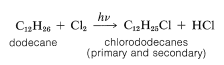This mixture of chlorododecanes is used to alkylate benzene, thereby giving a mixture of isomeric dodecylbenzenes, called detergent alkylate: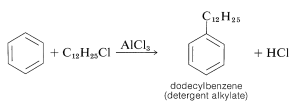Sulfonation of the detergent alkylate gives exclusively the 4-dodecylbenzenesulfonic acids, which with sodium hydroxide form water-soluble dodecylbenzenesulfonates: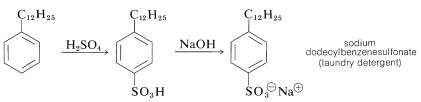In many countries it is prohibited by law to market detergents of this type, which have highly branched alkyl groups. The reason is that quaternary carbons and, to a lesser extent, tertiary carbons are not degraded readily by bacteria in sewage treatment plants:## Hydrogen Exchange

It is possible to replace the ring hydrogens of many aromatic compounds by exchange with strong acids. When an isotopically labeled acid such as $$\ce{D_2SO_4}$$ is used, this reaction is an easy way to introduce deuterium. The mechanism is analogous to other electrophilic substitutions:Perdeuteriobenzene$$^3$$ can be made from benzene in good yield if a sufficiently large excess of deuteriosulfuric acid is used. Deuteration might appear to be competitive with sulfonation, but deuteration actually occurs under much milder conditions.

## Aromatic Substitution by Electrophilic Metalation

Because metals are electropositive elements they can be considered potential electrophiles. Their reactions with arenes have been investigated most thoroughly for mercury. Benzene can be substituted with $$\ce{HgX}^\oplus$$ derived from a mercuric salt, $$\ce{HgX_2}$$, in the presence of an acid catalyst. The salt most commonly used is mercuric ethanoate, $$\ce{Hg(OOCCH_3)_2}$$. The catalyst is considered to function by assisting the generation of the active electrophile, $$\ce{HgX}^\oplus$$. Other metals that may be introduced directly into an aromatic ring in this manner include thallium and lead.$$^3$$The prefix per, as in perdeuterio- or perfluoro-, means that all the hydrogens have been replaced with the named substituent, $$\ce{D}$$ or $$\ce{F}$$. Perhydro means saturated or fully hydrogenated.